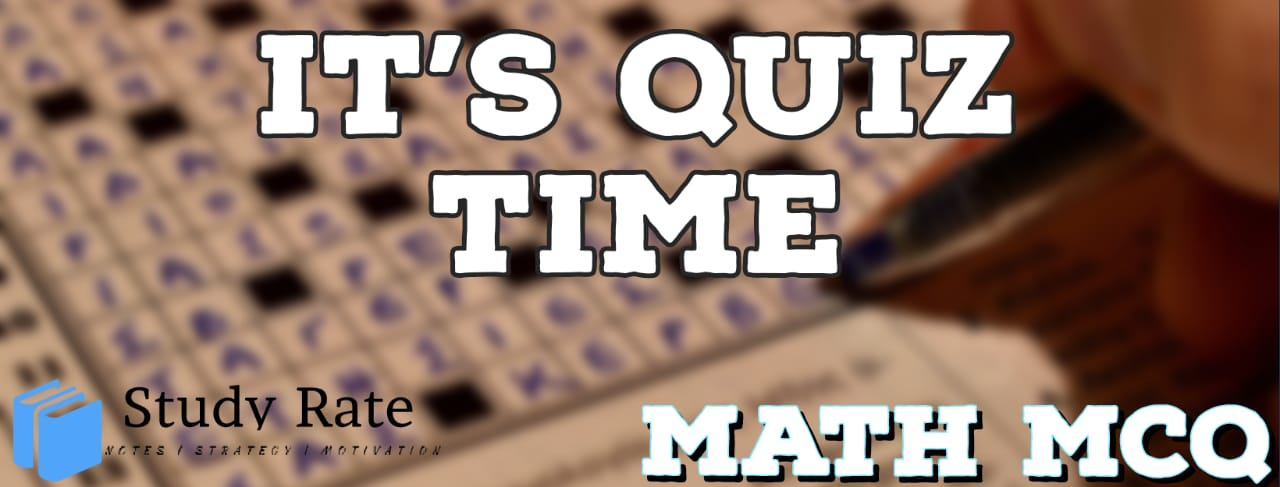## CBSE Class 10 Maths Chapter 12 – Area Related to Circles MCQ Quiz

Free PDF Download of CBSE Class 10 Maths Chapter 12 – Area Related to Circles Multiple Choice Questions with Answers. MCQ Questions for Class 10 Maths with Answers was Prepared Based on Latest Exam Pattern. Students can solve Class 10 Maths Area Related to Circles MCQs with Answers to know their preparation level

CBSE Board Class 10 Maths Chapter 12- Areas related to Circles Objective Questions

1. Find the area of the shaded region where ABC is a quadrant of radius 5cm and a semicircle is drawn with BC as the diameter2. Area of the shaded portion in the following figure is equal to area of3. There is a circular swimming pool with center O. The radius of pool is 5 m. There are 2 points on the wall of the pool separated by distance of 7 m. These 2 points are named A and B. A rope is attached between A and B. This rope separates the shallow section of pool from deep section of pool. The shallow section is the smaller section. Which of following statements are true?4. Radius of the outer circle is 18 cm and the radius of the inner circle is 7 cm. What is the area of the region between the outer and the inner circles?

5. There is a circle of diameter 10 cm. A chord of length 6 cm is drawn inside the circle. What is the distance between the centre and this chord in cm?

6. If the circumference of a circle and the perimeter of a square are equal, then

7. If the perimeter of a circle is equal to that of a square, then the ratio of their areas is:

8. It is proposed to build a single circular park equal in area to the sum of areas of two circular parks of diameters 16 m and 12 m in a locality. The radius of the new park would be

9. Find the area of the shaded region10. The radius of a circle whose circumference is equal to the sum of the circumferences of the two circles of diameters 36 cm and 20 cm is